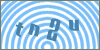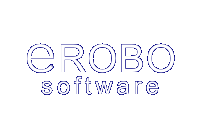Products
ServicesScripts: Cplus :: C++ string functions :: Library Article #4Developer's SectionConvert a Signed / Unsigned Binary number to Integer
By: Erobo Team Member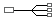Hire a Developer for Related Work / Installation | \$55 hr
 Rating: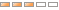| Rate It: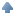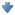Average Votes: (1371) Favorites:this library article shows how to convert a binary number to an integer.

The following two methods can be used to convert a signed / unsigned binary number to an integer: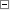Code Snippet 1//>>>>>>>>>>>>>>>>>>Converter<<<<<<<<<<<<<<<<<<<<<<

// a simple method to convert to decimal

int to_integer(string binary)
{

for(int i = 0; i < binary.size(); i++)
{
if(binary[binary.size() - 1 - i] == '1')
answer +=  pow(2 , i) * 1;
}
}

// convert signed number to integer
int to_integer_s(string binary)
{
string comm1;

if(binary == '1')
{
for(int i = 0; i < binary.size(); i++)
{
if(binary[binary.size() - 1 - i] == '0')
comm1 = "1" + comm1;

else{ comm1 = "0" + comm1;}
}

if(comm1[comm1.size() - 1] == '0' )
{
comm1[comm1.size() - 1] = '1';
}
else
{
int i = 0;

while(comm1[comm1.size() - 1 - i] == '1')
{
comm1[comm1.size() - 1 - i] = '0';
i++;
}
comm1[comm1.size() - 1 - i] = '1';
}
}

int answer = -1 * to_integer(comm1);See other Scripts in C++ string functionsSubmit Your Scripts: System Message:Your C++ script has been sent. Please allow 48 hours for processing.If you would like to have your C++ scripts published in this section please fill outthe form below:
 [ Refresh Image ]